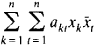# Hermitian Form

## Hermitian form

[er′mish·ən ′fȯrm]
(mathematics)
A polynomial in n real or complex variables where the matrix constructed from its coefficients is Hermitian.
More generally, a sesquilinear form g such that g (x,y) = g (y,x)for all values of the independent variables x and y, where g (x,y)is the image of g (x,y) under the automorphism of the underlying ring.
McGraw-Hill Dictionary of Scientific & Technical Terms, 6E, Copyright © 2003 by The McGraw-Hill Companies, Inc.
The following article is from The Great Soviet Encyclopedia (1979). It might be outdated or ideologically biased.

## Hermitian Form

an expression of the typewhere akt = ātk (ā is the complex conjugate of a). A matrix constructed from the coefficients of a Hermitian form is said to be Hermitian, as is a linear transformation that is defined by a Hermitian matrix. In 1854, C. Hermite investigated the representation of whole numbers by Hermitian forms for integral values of the arguments. The theory of Hermitian forms is in many respects similar to the theory of quadratic forms.

The Great Soviet Encyclopedia, 3rd Edition (1970-1979). © 2010 The Gale Group, Inc. All rights reserved.
References in periodicals archive ?
The quaternion algebra is one of the most important and well-studied objects in mathematics and physics; and it has natural Hermitian form which induces Euclidean metric .
A functional ([dot], [dot]) : X x X [right arrow] K is said to be a Hermitian form on X if
This is a real form (the group Sp(2) can be also defined as the subgroup of GL(2, H) which preserves the Hermitian form (x, y) = [[summation].sup.2.sub.i=1] [[bar.x].sub.i][y.sub.i], where [x.sub.i], [y.sub.i], [member of] H and [[bar.x].sub.i] is the quaternionic conjugate of [x.sub.i]) of Sp(4, C), called Sp(2) (or sometimes USp(4)).
The Strominger system admits a conformally balanced Hermitian form on a three-dimensional compact complex manifold M, a nowhere vanishing holomorphic (3,0)-form, and a HYM connection on a vector bundle E over this manifold.
[not equal to] 2, D a division k-algebra with center K = k([square root of (a)]) and involution J of second kind, non-trivial on K and h a non-degenerate hermitian form of rank n with respect to J and with values in D.
A hermitian form over (D,[sigma]) is a pair (V,h) where V is a D-vector space and h is a map h : V x V [right arrow] D which is [sigma]-sesquilinear in the first argument, D-linear in the second argument and which satisfies
In this section, we follow the exposition given in  in order to realize [N.sub.[omega]] := C [x.sub.[omega]] [C.sup.n] as a central extension of the Heisenberg group [H.sub.2n+1] := R [x.sub.Tm[omega]] [C.sup.n], where [omega](z, [omega]) denotes the standard Hermitian form on [C.sup.n].
where g is a Hermitian form of signature (2,2) and SU(2,2) is defined by
Ge, "The weight distribution of a class of cyclic codes related to Hermitian forms graphs," IEEE Transactions on Information Theory, vol.
His account relies on the basic results in the linear representations of finite groups in quadratic, symplectic, and Hermitian forms and in involutions over simple algebras, which are outlined, mainly without proofs, in the first chapter.
10, (1.1)], which relates hermitian forms over a quaternion algebra with canonical involution--the unique symplectic involution on a quaternion algebra--to quadratic forms over the center.

Site: Follow: Share:
Open / Close